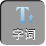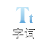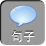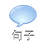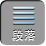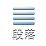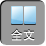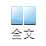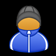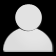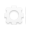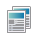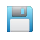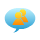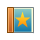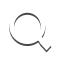-AA+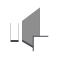1.等差数列｛ a n｝中， a 1+ a 2+ a 3= -24a 18+ a 19+ a 20= 78，则此数列前 20项和等于()

A160

B180

C200

D220

【考场错解】知通项公式 a n= a 1( n -1) d.a 1d来描述 a 2a 3a 18a 19a 20a 1d，再利用等差数列求和，选 C.

【专家把脉】此方法同样可求得解，但解法大繁，花费时间多，计算量大故而出错，应运用数列的性质求解就简易得多

【对症下药】 B

1.已知数列{ a n}的各项都是正数,且满足: a0= 1, a n+ 1=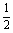a n·( 4 - a n), nN.

( 1)证明 a na n+ 12, nN.

( 2)求数列{ a n}的通项公式 a n.

【考场错解】 用数学归纳法证明：

1a．当 n= 1时， a0= 1, a 1=a04 - a0）=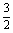,∴ a0a 12,命题正确.

b．假设 n= k时有 a k -1a k2.

n= k+ 1时，

a k - a k+ 1=a k -1( 4 - a k -1)-a k( 4 - a k)

= 2( a k -1 - a k)-( a k -1 - a k)( a k -1+ a k)

=( a k -1 - a k)( 4 - a k -1 - a k)

a k -1 - a k0. 4 - a k -1 - a k0,

a k - a k -10.

a k -1=a k( 4 - a k)=[ 4 -( a k -2) 2]＜ 2.

n= k+ 1时命题正确.

ab知对一切 nN时有 a na n+ 12.

( 2) a n+ 1=a n( 4 - a n)=[ -( a n -2) 2+ 4].

2( a n+ 1 -2)= -( a n -2) 2

a n+ 1 -2=( a n -2) 2

b n= a n -2,

b n=-() 1+ 2+…+ 2 n -1·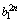b n=-() 2 n+ 2 n -1.

a n= 2 -() 2 n+ 2 n -1.

[专家把脉]在（Ⅱ）问中求 b n的通项时，运用叠代法最后到 b0而不是 b 1.

[对症下药]（Ⅰ）同上，方法二：用数学归纳法证明：

a.n= 1时， a0= 1, a 1=a0( 4 - a0)=,

0a0a 12;

b.假设 n= k时有 a k -1a k2成立，

f (x)=x( 4 - x),

f (x)在[ 02]上单调递增，

∴由假设有： f( a k -1)＜ f( a k)＜ f( 2),a k -1( 4 - a k -1)＜a k( 4 - a k)× 2( 4-2),

( 2)下面来求数列的通项： a n+ 1=a n( 4 - a n)=[ -( a n -2) 2+ 4],

b n= a n -2,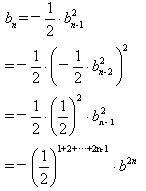b n= -1,所以 b n=-() 2 n -1,即 a n= 2+ b n= 2 -() 2 n -1